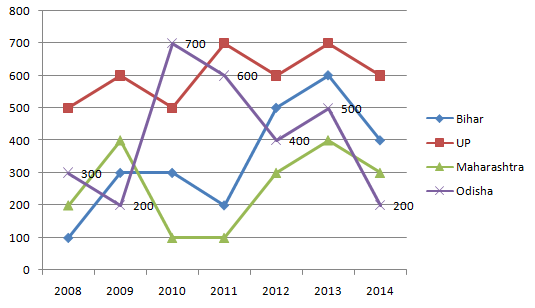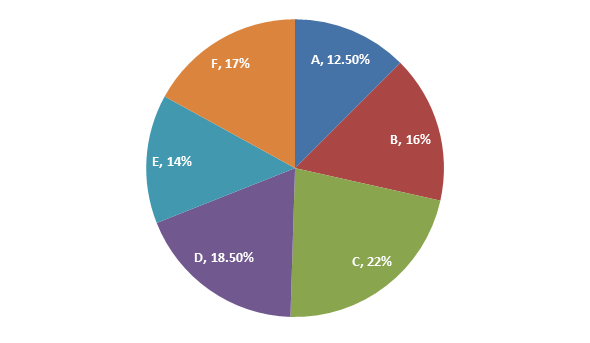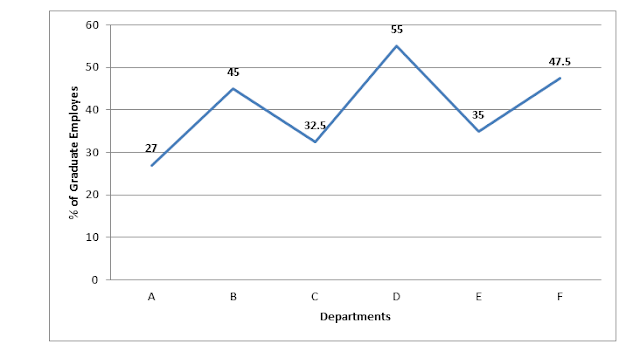## Data Interpretation Practice Questions for Bank Exams

Data Interpretation Practice Questions
Directions (1 – 5): Study the given chart and table carefully to answer the given questions. The graph shows the production of Rice, Maize, Pulses and Wheat in six different years.1. The number of persons who died in train accidents in 2013 is how much percent more than the number of persons who died in the train accident in 2011?
a) 143.5%
b) 137.5%
c) 37.5%
d) 127.5%
e) 147.5%
2. What is the average of the number of persons who died in train accidents in 2008 in all states together?
a) 182
b) 290
c) 275
d) 284
e) 307
3. In which state is the number of persons who died in the train accidents the maximum during the given period?
a) Odisha
b) UP
c) Bihar
d) Only a) and b)
e) Maharashtra
4. What is the difference between the number of train accidents in 2014 and that in 2012?
a) 5
b) 6
c) 7
d) 8
e) 9
5. What is the ratio of the number of persons who died in train accidents in 2010 to that in 2014?
a) 8 : 7
b) 10 : 9
c) 12 : 11
d) 14 : 13
e) 16 : 15
Directions (6 – 10): The given pie-chart shows the percentage distribution of employees among different departments of a Company and the line graph shows the percentage of graduate employees among them. Answer the following questions based on these graphs.
(Total number of employees in the Company is 8000)6. What is the total number of graduate employees working in Department A ?
a) 540
b) 270
c) 135
d) 1080
e) 730
7. What is the total number of employees working in the Company who are non-graduates?
a) 3780
b) 3940
c) 4360
d) 4730
e) 5730
8. The total number of graduate employees working in Department E is what percent of the total number of employees of the Company?
a) 7.2%
b) 6.4%
c) 4.9%
d) 4.3%
e) None of these,
9. The total number of graduate employees working in Department D is approximately what percent more or less than the total number of non-graduate employees working in that department?
a) 18% more
b) 22% more
c) 24% less
d) 27% less
e) 32% less
10. What is the average number of graduate employees working in the Company in all departments together?
a) 535
b) 545
c) 555
d) 565
e) 575
Solutions:
1. C) The number of people who died in train accidents in 2013 = 400 + 500 + 600 + 700 = 2200
The number of people who died in train accidents in 2011 = 100 + 200 + 600 + 700 = 1600
So, required % = 2200 – 1600 x 100  /  1600 = 37.5%
2. C) Average number of people who died in train accidents in all states in 2008 = 1/4 × (100 + 200 + 300 + 500) = 1100/4 = 275
3. B) The number of deaths in train accidents in Bihar = 100 + 300 + 300 + 200 + 500 + 600 + 400 = 2400
Similarly, in UP = 500 + 600 + 500 + 700 + 600 + 700 + 600 = 4200
In Maharashtra = 200 + 400 + 100 + 100 + 300 + 400 + 300 = 1800
In Odisha = 300 + 200 + 700 + 600 + 400 + 500 + 200 = 2900
In UP the number of people who died in train accidents is the maximum.
4. D) The number of train accidents in 2014 = 200 × 18/100 = 36
The number of train accidents in 2012 = 200 × 14/100 = 28
So, required difference = 36 28 = 8
5. E) The ratio of the number of deaths in 2010 to that in 2014 = (100 + 300 + 500 + 700) : (200 + 300 + 400 + 600) = 1600 : 1500 = 16 : 15
6. B) Total number of graduate employees working in Department A = 8000 × 12.5/100 × 27/100 = 8 × 1.25 × 27 = 270
7. D) Total number of non – graduate employees = [8000/(100 × 100)][ 12.5 × 73 + 16 × 55 + 22 × 67.5 + 18.5 × 45 + 14 × 65 + 17 × 52.5] = 0.8(912.5 + 880 + 1485 + 832.5 + 910 + 892.5} = 0.8 × 5912.5 = 4730
8. C) Total number of graduate employees working in Department E = 8000 × 14/100 × 35/100 = 392
Therefore Required Percentage = 392/8000 × 100 = 4.9%
9. B) Total number of graduate employees working in Department D = 8000 × 18.5/100 × 55/100 = 814
Total number of non – graduate employees working in Department D = 8000 × 18.5/100 × 45/100 = 666
Therefore required percentage = (814 – 666)/666 × 100 = 14800/666 = 22.22% ≈ 22% more
10. B) Total number of non – graduate employees = 4730 (see explanation no. 9)
Total number of graduate employees = 8000 – 4730 = 3270
Therefore, Required average =3270/6 = 545